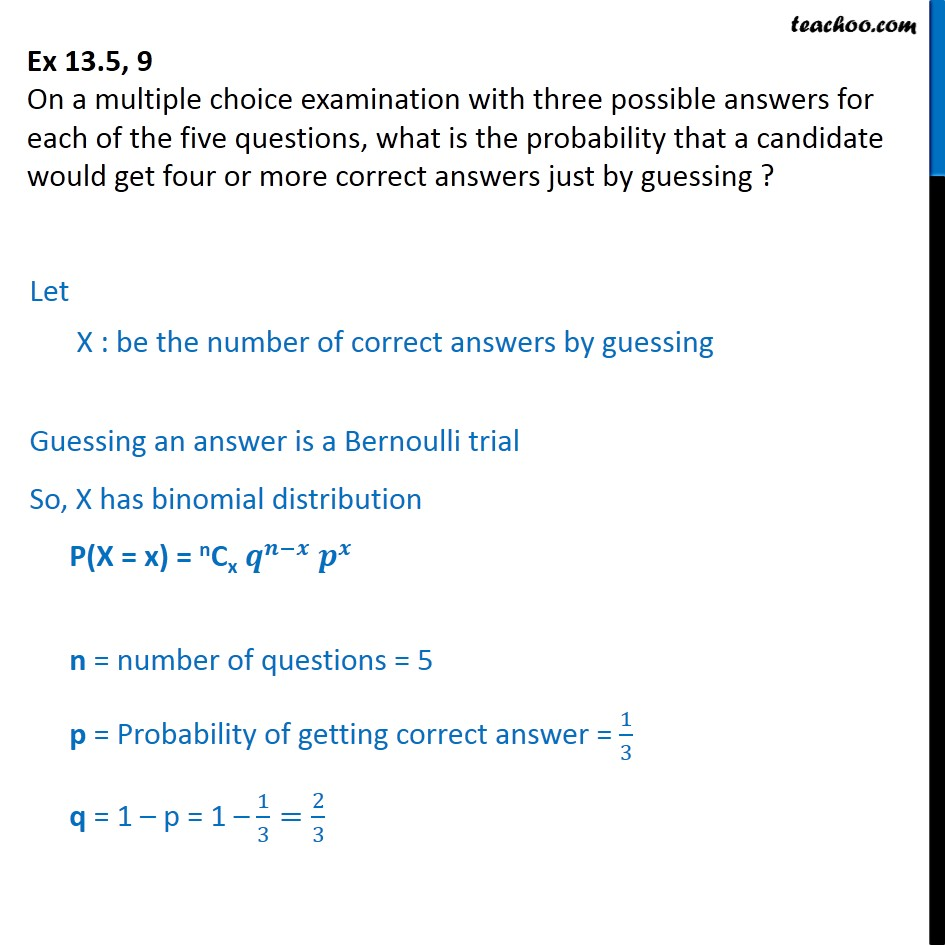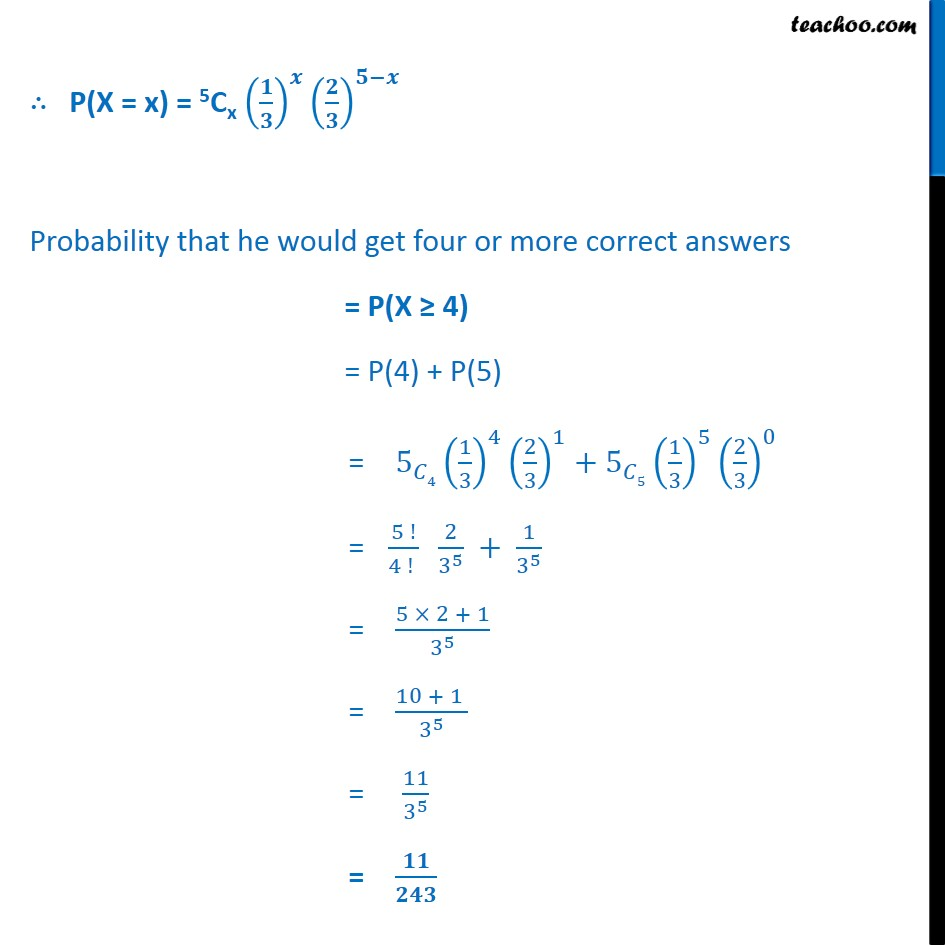Bernoulli Trial

Chapter 13 Class 12 Probability
Serial order wiseLearn in your speed, with individual attention - Teachoo Maths 1-on-1 Class

### Transcript

Question 9 On a multiple choice examination with three possible answers for each of the five questions, what is the probability that a candidate would get four or more correct answers just by guessing ? Let X : be the number of correct answers by guessing Guessing an answer is a Bernoulli trial So, X has binomial distribution P(X = x) = nCx 𝒒﷮𝒏−𝒙﷯ 𝒑﷮𝒙﷯ n = number of questions = 5 p = Probability of getting correct answer = 1﷮3﷯ q = 1 – p = 1 – 1﷮3﷯= 2﷮3﷯ ∴ P(X = x) = 5Cx 𝟏﷮𝟑﷯﷯﷮𝒙﷯ 𝟐﷮𝟑﷯﷯﷮𝟓−𝒙﷯ Probability that he would get four or more correct answers = P(X ≥ 4) = P(4) + P(5) = 5﷮𝐶4﷯ 1﷮3﷯﷯﷮4﷯ 2﷮3﷯﷯﷮1﷯+ 5﷮𝐶5﷯ 1﷮3﷯﷯﷮5﷯ 2﷮3﷯﷯﷮0﷯ = 5 !﷮4 ! ﷯ 2﷮ 3﷮5﷯﷯ + 1﷮ 3﷮5﷯﷯ = 5 × 2 + 1﷮ 3﷮5﷯﷯ = 10 + 1 ﷮ 3﷮5﷯﷯ = 11﷮ 3﷮5﷯﷯ = 𝟏𝟏﷮𝟐𝟒𝟑﷯